Tamilnadu State Board New Syllabus Samacheer Kalvi 8th Maths Guide Pdf Chapter 4 Life Mathematics Ex 4.3 Text Book Back Questions and Answers, Notes.

## Tamilnadu Samacheer Kalvi 8th Maths Solutions Chapter 4 Life Mathematics Ex 4.3

This calculator computes any of the values in the half-life formula given the rest values.

Question 1.
Fill in the blanks:
(i) The compound interest on ₹ 5000 at 12% p.a for 2 years, compounded annually is ________ .
₹ 1272
Hint:
Compound Interest (CI) formula is
CI = Amount – Principal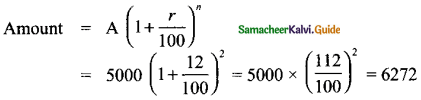∴ 6272 – 5000 = ₹ 1272(ii) The compound interest on ₹8000 at 10% p.a for 1 year, compounded half yearly is ________ .
₹ 820
Hint:
Compound interest (CI) = Amount – Principal
Amount = p $$\left(1+\frac{r}{100}\right)^{2 n}$$ [2n as it is compounded half yearly]
r = 10% p.a, for half yearly r = $$\frac{10}{2}$$ = 5
∴ A = 8000 $$\left(1+\frac{5}{100}\right)^{2 \times 1}$$ = 8000 × $$\left(\frac{105}{100}\right)^{2}$$ = 8820
CI = Amount – principal = 8820 – 8000 = ₹ 820

(iii) The annual rate of growth in population of a town is 10%. If its present population is 26620, then the population 3 years ago was ________ .
₹ 20,000
Hint:
Rate of growth of population r = 10%
Present population = 26620
Let population 3 years ago be x
∴ Applying the formula for population growth which is similar to compound interest,The population 3 years ago was ₹ 20,000(iv) If the compound interest is calculated quarterly, the amount is found using the formula ________ .
A = $$P\left(1+\frac{r}{400}\right)^{4 n}$$
Hint:
Quarterly means 4 times in a year.
∴ The formula for compound interest is
A = $$P\left(1+\frac{r}{400}\right)^{4 n}$$

(v) The difference between the C.I and S.I for 2 years for a principal of ₹ 5000 at the rate of interest 8% p.a is ________ .
₹ 32
Hint:
Difference between S.I & C.I is given by the formula
CI – SI = $$\left(\frac{r}{100}\right)^{2}$$
Principal (P) = 5000. r = 8% p.a
∴ CI – SI = 5000$$\left(\frac{8}{100}\right)^{2}$$ = 5000 × $$\left(\frac{8}{100}\right)^{2}$$ × $$\left(\frac{8}{100}\right)^{2}$$ = ₹ 32

CBSE Class 10 Maths formulas and equations are available chapter wise.

Question 2.
Say True or False.
(i) Depreciation value is calculated by the formula, $$P\left(1-\frac{r}{100}\right)^{n}$$.
True
Hint:
Depreciation formula is $$P\left(1-\frac{r}{100}\right)^{n}$$

(ii) If the present population ola city is P and it increases at the rate of r% p.a, then the population n years ago would be $$P\left(1-\frac{r}{100}\right)^{n}$$
False
Hint:
Let the population ‘n’ yrs ago be ‘x’
∴ Present popuLation (P) = x × $$\left(1+\frac{r}{100}\right)^{n}$$
∴ x = $$\frac{P}{\left(1+\frac{r}{100}\right)^{n}}$$

(iii) The present value of a machine is ₹ 16800. It depreciates at 25% p.a. Its worth after 2 years is ₹ 9450.
True
Hint:
Present value of machine = ₹ 16800
Depreciation rate (n) = 25%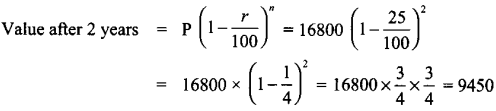(iv) The time taken for ₹ 1000 to become ₹ 1331 at 20% p.a, compounded annually is 3 years.
False
Hint:
Pnncipal money = 1000
rate of interest = 20%
Amount = 1331, applying in formula we get(v) The compound interest on ₹ 16000 for 9 months at 20% p.a, compounded quarterly is ₹ 2522.
True
Hint:
Principal (P) = 16000
n = 9 months = $$\frac{9}{12}$$ years
r = 20% p.a
For compounding quarterly, we have to use below formula,
Amount(A) = P × $$\left(1+\frac{r}{100}\right)^{4 n}$$
Since quarterly we have to divide ‘r’ by 4∴ Interest A – P = 18522 – 16000 = 2522 (True)Question 3.
Find the compound interest on ₹ 3200 at 2.5 % p.a for 2 years, compounded annually.
Principal (P) = ₹ 3200
r = 2.5% p.a
n = 2 years comp. annually
∴ Amount (A) = $$\left(1+\frac{r}{100}\right)^{n}$$
= 3200 $$\left(1+\frac{25}{100}\right)^{2}$$
= 3200 × (1.025)2 = 3362
Compound interest (CI) = Amount – Principal
= 3362 – 3200 = 162

Question 4.
Find the compound interest for 2$$\frac { 1 }{ 2 }$$ years on ₹ 4000 at 10% p.a, if the interest is compounded yearly.
Principal (P) = ₹ 4000
r = 10 %p.a
Compounded yearly
n = 2$$\frac { 1 }{ 2 }$$ years. Since it is of the form a $$\frac{b}{c}$$ years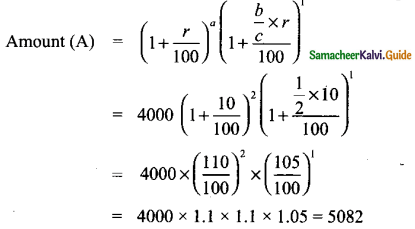∴ CI = Amount – principal = 5082 – 4000 = 1082Question 5.
A principal becomes ₹ 2028 in 2 years at 4% p.a compound interest. Find the principal.
n = 2 years
r = rate of interest = 4% p.a
Amount A = ₹ 2028Question 6.
In how many years will ₹ 3375 become ₹ 4096 at 13 % p.a if the interest is compounded half-yearly?
Principal = ₹ 3375
Amount = ₹ 4096
r = 13$$\frac{1}{3}$$%p.a = $$\frac{40}{3}$$%p.aLet no. of years be n
for compounding half yearly, formula is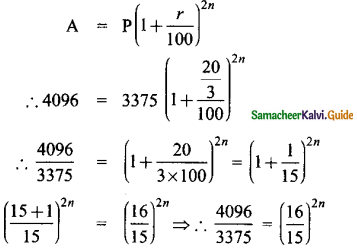Taking cubic root on both sides,Question 7.
Find the CI on ₹ 15000 for 3 years if the rates of interest are 15%, 20% and 25% for the I, II and III years respectively.
Principal (P) = ₹ 15000
rate of interest 1 (a) = 15% for year I
rate of interest 2 (b) = 20% for year II
rate of interest 3 (c) = 25% for year III
Formula for amount when rate of interest is different for different years isSubstituting in the above formula, we get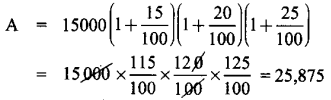∴ Compound Interest(CI) = A – P = 25,875 – 15,000 = ₹ 10,875
CI = ₹ 10.875

Question 8.
Find the difference between C.I and S.I on ₹ 5000 for 1 year at 2% p.a, if the interest is compounded half yearly.
Principal (P) = ₹ 5000
time period (n) = 1 yr.
Rate of interest (r) = 2%p.a
for half yearly r = 1%
Difference between CI & SI is given by the formula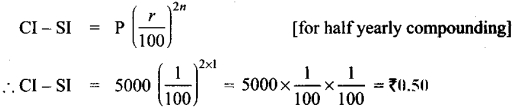Question 9.
Find the rate of interest if the difference between C.I and S.I on ₹ 8000 compounded annually for 2 years is ₹ 20.
Principal (P) = ₹ 8000
time period (n) = 2 yrs.
rate of interest (r) = ?
Difference between CI & SI is given by the formula
CI – SI = $$p\left(1+\frac{r}{100}\right)^{n}$$
Difference between CI & SI is given as 20
∴ 20 = 8000 × $$\left(\frac{r}{100}\right)^{2}$$
∴ $$\left(\frac{r}{100}\right)^{2}=\frac{20}{8000}=\frac{1}{400}$$
Taking square root on both sides
$$\frac{r}{100}=\sqrt{\frac{1}{400}}=\frac{1}{20}$$
∴ r =  = 5 %

Question 10.
Find the principal if the difference between C.I and S.l on it at 15% p.a for 3 years is ₹ 1134.
Rate of interest (r) = 15% p.a
time period (n) = 3 years
Difference between CI & SI is given as 1134
Principal = ? → required to find
Using formula for difference
Simple Interest SI = $$\frac{P n r}{100}$$
Compound Interest CI = p(1 + i)n – p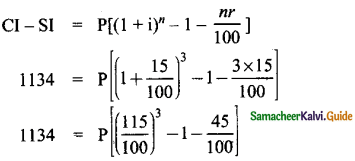1134 = P[(1.15)3 – 1 – 0.45] = P(1.52 – 1.45) = P (0.07)
∴ p = $$\frac{1134 \times 100}{0.07 \times 100}=\frac{113400}{7}$$
P = ₹ 16200Objective Type Questions

Question 11.
The number of conversion periods in a year, if the interest on a principal is compounded every two months is _________ .
(A) 2
(B) 4
(C) 6
(D) 12
(C) 6
Hint:
Conversion period is the time period after which the interest is added to the principal. If principal is compounded every two months then in a year, there will be 6$$\left(\frac{12}{2}\right)$$ conversion periods.

Question 12.
The time taken for ₹ 4400 to become ₹ 4851 at 10%, compounded half yearly is _________ .
(A) 6 months
(B) 1 year
(C) 1$$\frac{1}{2}$$ years
(D) 2 years
(B) 1 year
Hint:
Principal = ₹ 4400
Amount = ₹ 4851
Rate of interest = 10% p.a
for half yearly, divide by 2,
r = $$\frac{10}{2}$$ = 5 %
Compounded half yearly, so the formula is
A = P$$P\left(1+\frac{r}{100}\right)^{2 n}$$
Substituting in the above formula, we get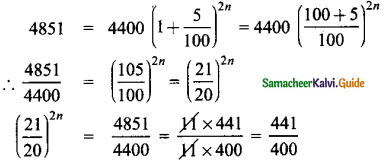Taking square root on both sides, we get
$$\left(\frac{21}{20}\right)^{2 n}=\left(\frac{21}{20}\right)^{2}$$
Equating power on both sides
∴ 2n = 2, n = 1Question 13.
The cost of a machine is ₹ 18000 and it depreciates at 16$$\frac{2}{3}$$% annually. Its value after 2 years will be _________ .
(A) ₹ 2000
(B) ₹ 12500
(C) ₹ 15000
(D) ₹ 16500
(B) ₹ 12500
Hint:
Cost of machine = 18000
Depreciation rate = 16$$\frac{2}{3}$$% = $$\frac{50}{3}$$%p.a
time period = 2 years
∴ As per depreciation formula,
Depriciated value = Original value $$\left(1-\frac{r}{100}\right)^{n}$$
Substituting in above formula, we get
Depreciated value after 2 yearsQuestion 14.
The sum which amounts to ₹ 2662 at 10% p.a in 3 years, compounded yearly is _________ .
(A) ₹ 2000
(B) ₹ 1800
(C) ₹ 1500
(D) ₹ 2500
(A) ₹ 2000
Hint:
Amount = ₹ 2662
rate of interest = 10 % p.a
Time period = 3 yrs. Compounded yearly
Principal (P) → required to find?Question 15.
The difference between compound and simple interest on a certain sum of money for 2 years at2%p.ais U. The sum of money is _________ .
(A) ₹ 2000
(B) ₹ 1500
(C) ₹ 3000
(D) ₹ 2500
CI – SI = $$P \times\left(1+\frac{r}{100}\right)^{n}$$
1 = p × $$\left(\frac{2}{100}\right)^{2}$$
∴ p = 1 × $$\left(\frac{100}{2}\right)^{2}$$ = 1 × (50)2 = ₹ 2500